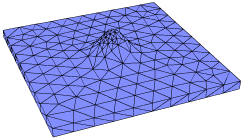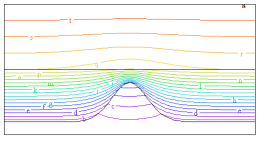﻿ Sample Problems > Usage > Mesh_Control > 3d_curvature

# 3d_curvature

Navigation:  Sample Problems > Usage > Mesh_Control >

# 3d_curvature{ 3D_CURVATURE.PDE

This problem demonstrates automatic mesh densification due to curvature and

proximity to small features.

The example consists of a three-layer heatflow problem.  The bottom layer contains

a hidden rise, or "dimple", that rises close to the base of the adjoining layer.

FlexPDE detects this dimple and automatically refines the computation mesh to

resolve the curvature of the tip.

It also detects the proximity of the dimple peak to the adjoining layer and refines the

mesh in that layer as well.

}

 title '3D Layer curvature resolution Test'   coordinates    cartesian3   select    paintregions   variables    Tp   definitions    long = 1    wide = 1    K  = 1    Q = 0    narrow = 0.2    z1 = 0    z2 = 0.1+0.3*exp(-(x^2+y^2)/narrow^2)    z3 = 0.5    z4= 1   initial values    Tp = 0.   equations    Tp:  div(k*grad(Tp)) + Q = 0   extrusion z = z1,z2,z3,z4boundaries

surface 1 value (Tp)=0

surface 4 value (Tp)=1

Region 1

layer 1 k=10

layer 3 k=5

start (-wide,-wide)

line to (wide,-wide) to (wide,wide) to (-wide,wide) to close

monitors

grid (x,z) on y=0

contour (Tp) on z=0.38  painted

plots

grid(x,y,z) on layer 1

grid (x,z) on y=0

grid(x,y) on surface 2

contour (Tp) on y=0 as "ZX Temp"

contour (Tp) on z=0.38  painted

end